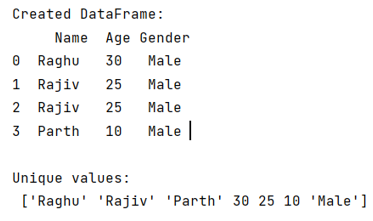# How to find unique values from multiple columns in pandas?

Given a Panadas DataFrame, we have to find the unique values from multiple columns in pandas.
Submitted by Pranit Sharma, on June 13, 2022

Pandas is a special tool that allows us to perform complex manipulations of data effectively and efficiently. Inside pandas, we mostly deal with a dataset in the form of DataFrame. DataFrames are 2-dimensional data structures in pandas. DataFrames consist of rows, columns, and the data. DataFrame can be created with the help of python dictionaries or lists but in the real world, CSV files are imported and then converted into DataFrames. Sometimes, DataFrames are first written into CSV files. Here, we are going to write a DataFrame into a CSV file.

A DataFrame may have a single or multiple columns, and these columns may have unique or similar values.

## Problem statement

Given a Panadas DataFrame, we have to find the unique values from multiple columns in pandas.

## Finding unique values from multiple columns in pandas

To find unique values in multiple columns, we will use the pandas.unique() method. This method traverses over DataFrame columns and returns those values whose occurrence is not more than 1 or we can say that whose occurrence is 1.

Syntax:

```pandas.unique(values)

# or
df['col'].unique()
```
Note

To work with pandas, we need to import pandas package first, below is the syntax:

```import pandas as pd
```

Let us understand with the help of an example,

## Python program to find unique values from multiple columns

```# Importing pandas package
import pandas as pd

# Creating a dictionary
d = {
'Name':['Raghu','Rajiv','Rajiv','Parth'],
'Age':[30,25,25,10],
'Gender':['Male','Male','Male','Male']
}

# Creating a DataFrame
df = pd.DataFrame(d)

# Display DataFrame
print("Created DataFrame:\n",df,"\n")

# Returning unique values from all the columns
result = pd.concat([df['Name'],df['Age'],df['Gender']]).unique()

# Display result
print("Unique values:\n",result)
```

### Output

The output of the above program is: